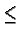CSE 5311 Fall 1999

Quiz #2

1.
(10 points.) Show how Quicksort can be made to run in O(n lg n) time in the worst case by making use of an order statistics algorithm.

2.
(10 points.) Consider the following variant of insertion sort: for 2in, to insert the key A[i] among AA[i-1], perform a binary search to find the correct position for A[i].

How many key comparisons would be performed in the worst case?